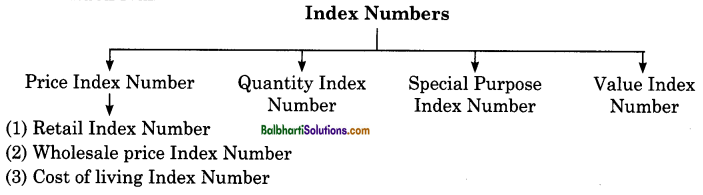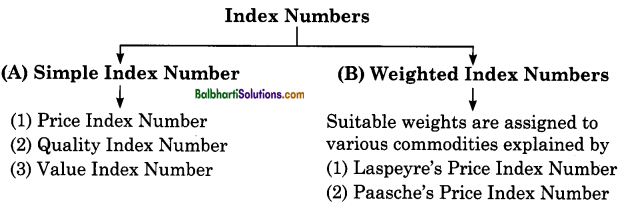By going through these Maharashtra State Board Class 12 Economics Notes Chapter 6 Index Numbers students can recall all the concepts quickly.

## Maharashtra State Board Class 12 Economics Notes Chapter 6 Index Numbers

Definition:
Spiegel defines Index Number as, “statistical measures designed to show the changes in a variable or a group of related variables with respect to time, geographical location, other characteristics such as income, profession, etc. ”

Hence, Index Numbers are :

• These are specialized forms of averages.
• These measures the net changes in a group of related variables like price, quantity, cost of living, etc.
• These are expressed in percentage without using the percentage sign.
• These are also known as economic barometers.
• They always refer to two periods, i.e. base year and the current year.
• Price Index Number P01 = $$\frac{p_{1}}{p_{0}}$$ x 100

where P01 = Price Index Number
p1 = Price of the commodity in the current year.
p0 = Price of the commodity in the base year.

Types of Index Numbers:Significance or Uses of Index Numbers:

• They help in formulating economic and business policies.
• They help in the study of economic trends and tendencies.
• They are considered as economic barometers as they measure economic changes over period of time.
• They help in the measurement of Inflation.
• They help in presenting financial data in real terms.
• They help in determining depreciation cost.
• They help the government to adjust national income.

Steps Involved in Construction of Index Numbers:

• Purpose of Index Number
• Selection of the base year
• Selection of commodities
• Selection of prices
• Selection of suitable averages
• Selection of formula
• Assigning proper weights.

Limitations of Index Numbers:
Although index numbers are very useful to business and industry they suffer from following
limitations:

• Bias in the data
• Based on samples
• Misuse of index numbers
• Defects in the formulae
• Changes in the economy
• Neglecting Qualitative changes
• Arbitrary weights
• Limited scope

Because of all these limitations, sometimes economists do not get desired results.

Different Methods of Constructing Index Numbers:
Following chart helps us to know different methods of constructing Index Numbers:Simple Index Number: It is constructed when all the items like food, clothing, transport, housing, etc. are given equal importance. There are three different methods of constructing it.

→ Price Index Number: It is constructed by using following formula: Po1 = $$\frac{\Sigma p_{1}}{\Sigma p_{0}}$$ × 100 where – p01 Price Index
Σp1 = Total of the current year prices of various commodities.
Σp0 = Total of base year prices of various commodities.

→ Quantity Index Number (Q01): It is calculated by using following formula :
Q01 = $$\frac{\Sigma q_{1}}{\Sigma q_{0}}$$ × 100
where, Σq1 = Sum total of current year quantities of commodities.
Σq0 = Sum total of base year quantities of commodities.

→ Value Index Number (V01): It is calculated by using following formula :
V01 = $$\frac{\Sigma p_{1} q_{1}}{\Sigma p_{0} q_{0}}$$ × 100
where, Ep1q1 = Sum total of product of prices and quantities of current year.
Ep0qo= Sum total of product of prices and quantities of base year.

Weighted Index Number: Under this method physical quantities are used as weights. Therefore prices themselves are weighted by quantities. We can take either the base year quantities or current year quantities as weights or the average of the two.

These index numbers are simple aggregative type with the fundamental difference that weights are assigned to various items included in the index. There are various methods of constructing weighted index numbers such as :

1. Laspeyres Price Index Number
2. Paasche’s Price Index Number

Laspeyre’s Price Index Number: This method was derived by Laspeyre’s, a German economist in 1871. In this method, weight are determined by quantities in the base year. He used the following
formula: P01 = $$\frac{\Sigma p_{1} q_{0}}{\Sigma p_{0} q_{0}}$$ x 100

where P01 = Price Index
p0 = Price of the base year
p0 = Price of the current year
q0 = Quantities of the base year
Σp1q0= Add all the products of p1 and q0
Σp0q0= Add all the products of p0 and q0

Paasche’s Price Index Number: This method was derived by Paasche, a German economist in 1874. In this method weight of the current year are used as base year. He used the following
formula: P01 = x 100

where P01 = Price of the current year
p0 = Price of the base year
p1 = Price of the current year
q1 = Quantities of the current year
Σp1q1 Add all the products of p1 and q1
Σp0q0= Add all the products of p0 and q1.